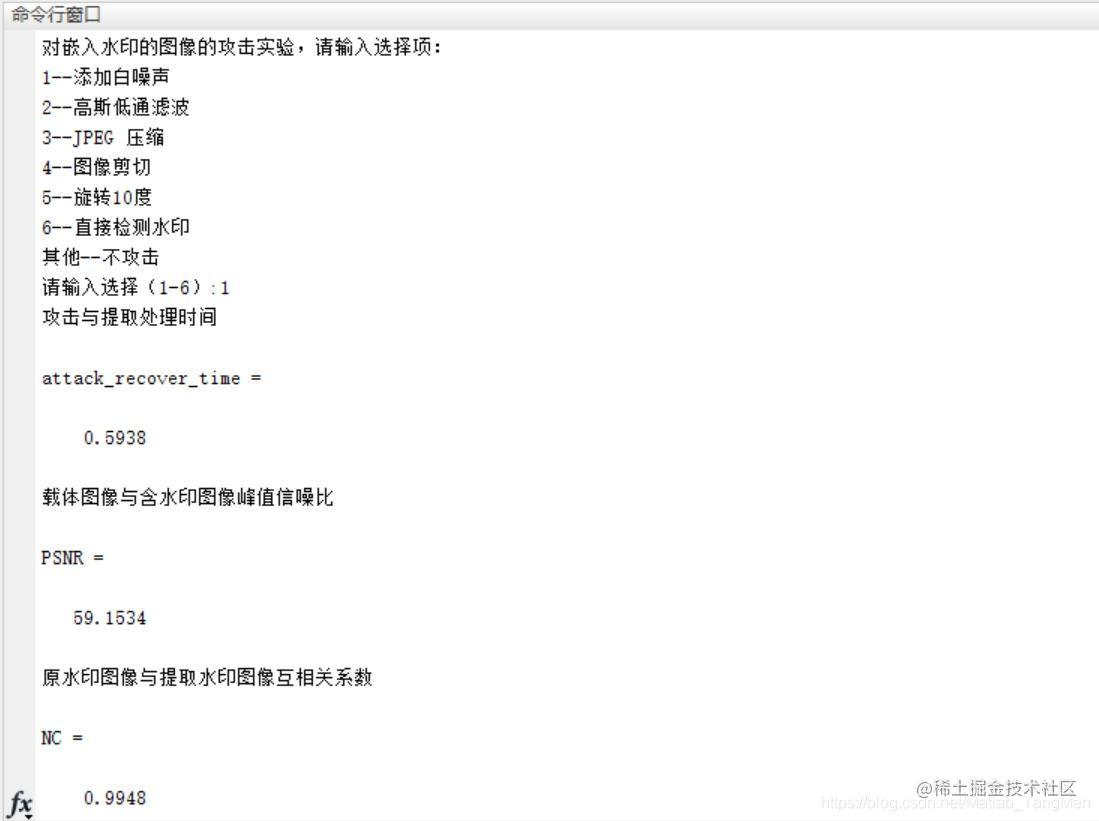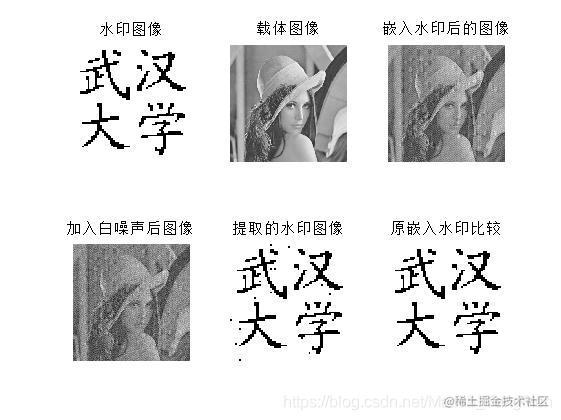# 【图像隐写】基于matlab DCT数字水印嵌入+检测+攻击（测试鲁棒性）【含Matlab源码 1133期】

## 一、简介

DCT变换的全称是离散余弦变换(Discrete Cosine Transform)，主要用于将数据或图像的压缩，能够将空域的信号转换到频域上，具有良好的去相关性的性能。DCT变换本身是无损的，但是在图像编码等领域给接下来的量化、哈弗曼编码等创造了很好的条件，同时，由于DCT变换时对称的，所以，我们可以在量化编码后利用DCT反变换，在接收端恢复原始的图像信息。DCT变换在当前的图像分析已经压缩领域有着极为广大的用途，我们常见的JPEG静态图像编码以及MJPEG、MPEG动态编码等标准中都使用了DCT变换。

1 一维DCT变换 一维DCT变换时二维DCT变换的基础，所以我们先来讨论下一维DCT变换。一维DCT变换共有8种形式，其中最常用的是第二种形式，由于其运算简单、适用范围广。我们在这里只讨论这种形式，其表达式如下：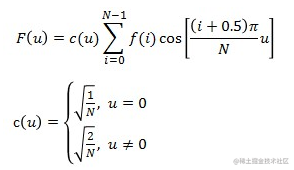其中，f(i)为原始的信号，F(u)是DCT变换后的系数，N为原始信号的点数，c(u)可以认为是一个补偿系数，可以使DCT变换矩阵为正交矩阵。

2 二维DCT变换 二维DCT变换其实是在一维DCT变换的基础上在做了一次DCT变换，其公式如下：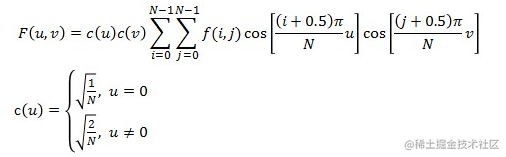由公式我们可以看出，上面只讨论了二维图像数据为方阵的情况，在实际应用中，如果不是方阵的数据一般都是补齐之后再做变换的，重构之后可以去掉补齐的部分，得到原始的图像信息，这个尝试一下，应该比较容易理解。 另外，由于DCT变换高度的对称性，在使用Matlab进行相关的运算时，我们可以使用更简单的矩阵处理方式：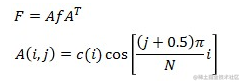## 二、源代码

``````%%%%%%%%%%%%%%%%%%%%%%%%%%%%%%%%%%%%%%%%%%%%%
%%%% 数字水印嵌入、攻击、检测             %%%
%%%%    %%%
%%%%%%%%%%%%%%%%%%%%%%%%%%%%%%%%%%%%%%%%%%%%%
clear all;
clc;
start_time=cputime;
%%%%%%%%%%%% 读取水印图像 %%%%%%%%
I=rgb2gray(I);
I=double(I)/255;
I=ceil(I);
%%%%%%%%%%显示水印图像%%%%%%%%%%%%%
figure(1);
subplot(2,3,1);
imshow(I),title('水印图像')
dimI=size(I);
rm=dimI(1);cm=dimI(2);
%%%%%%%%%%%%%%%5  以下生成水印信息 %%
mark=I;
alpha=50,

psnr_cover=double(a0);
subplot(2,3,2),imshow(a0,[]),title('载体图像');
[r,c]=size(a0);
cda0=blkproc(a0,[8,8],'dct2');
%%%%%%%%%%%%%%%%%%%%% 嵌入 %%%%%%%%%%
cda1=cda0;   % cda1 = 256_256
for i=1:rm  % i=1:32
for j=1:cm  % j=1:32
x=(i-1)*8;y=(j-1)*8;
if mark(i,j)==1
k=k1;
else
k=k2;
end
cda1(x+1,y+8)=cda0(x+1,y+8)+alpha*k(1);
cda1(x+2,y+7)=cda0(x+2,y+7)+alpha*k(2);
cda1(x+3,y+6)=cda0(x+3,y+6)+alpha*k(3);
cda1(x+4,y+5)=cda0(x+4,y+5)+alpha*k(4);
cda1(x+5,y+4)=cda0(x+5,y+4)+alpha*k(5);
cda1(x+6,y+3)=cda0(x+6,y+3)+alpha*k(6);
cda1(x+7,y+2)=cda0(x+7,y+2)+alpha*k(7);
cda1(x+8,y+1)=cda0(x+8,y+1)+alpha*k(8);

end
end

%%%%%% 攻击实验 测试鲁棒性 %%%%%%%%%%%
disp('对嵌入水印的图像的攻击实验，请输入选择项：');
disp('1--添加白噪声');
disp('2--高斯低通滤波');
disp('3--JPEG 压缩');
disp('4--图像剪切');
disp('5--旋转10度');
disp('6--直接检测水印');
disp('其他--不攻击');
d=input('请输入选择（1-6）:');
start_time=cputime;

figure(1);
switch d
case 6
subplot(2,3,4);
imshow(a1,[]);
title('未受攻击的含水印图像');
M1=a1;
case 1
WImage2=a1;
noise0=20*randn(size(WImage2));
WImage2=WImage2+noise0;
subplot(2,3,4);
imshow(WImage2,[]);
title('加入白噪声后图像');
M1=WImage2;
M_1=uint8(M1);
imwrite(M_1,'whitenoise.bmp','bmp');

case 2
WImage3=a1;
H=fspecial('gaussian',[4,4],0.2);
WImage3=imfilter(WImage3,H);
subplot(2,3,4);
imshow(WImage3,[]);
title('高斯低通滤波后图像');
M1=WImage3;
M_1=uint8(M1);
imwrite(M_1,'gaussian.bmp','bmp');

case 4
WImage4=a1;
WImage4(1:64,1:512)=512;
%WImage4(224:256,1:256)=256;
%WImage4(1:256,224:256)=256;
%WImage4(1:256,1:32)=256;
WImage4cl=mat2gray(WImage4);
figure(2);
subplot(1,1,1);
%subplot(2,3,4);
imshow(WImage4cl);
title('部分剪切后图像');
figure(1);
M1=WImage4cl;
%M_1=uint8(M1);
%imwrite(M_1,'cutpart.bmp','bmp');
function N=nc(mark_get,mark_prime)
mark_get=double(mark_get);
mark_prime=double(mark_prime);
if size(mark_get)~=size(mark_prime)
error('Input vectors must  be the same size!')
else
[m,n]=size(mark_get);
fenzi=0;
fenmu=0;
for i=1:m
for j=1:n
fenzi=fenzi+mark_get(i,j)*mark_prime(i,j);
fenmu=fenmu+mark_prime(i,j)*mark_prime(i,j);
end
end

## 三、运行结果